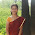## Thursday, March 28, 2013

### group obs almost evenly and calculate cumulative stats in SAS and R

The input is 99 records, the requirement is to split it into 10 groups as evenly as possible(10 records each for first 9 groups, and 9 records in the last group). And then get the cumulative sum/mean in each group.

The output is like:

In SAS, a loop is required to do this because of cumulative sum.

data test;

i=1;

p=.99;

output;

do i=2 to 98;

p=.8;

output;

end;

i=99;

p=.2;

output;

run;

proc print data=test;

run;

* if use proc rank, it cannot group data evenly because of ties;

proc rank data=test out=t group=10;

var p;

ranks rank;

run;

proc print data=t;

run;

* so need to mannuly do it;

%let dsid=%sysfunc(open(test));          *open the file;

%let nobs=%sysfunc(attrn(&dsid,nobs));   *count the obs in file; %let ngroup=10; %let overall_pct=.5; %put &nobs;

* data n_per_group only has one obs;

data n_per_group;

n_per_grp=int(&nobs/&ngroup.);  * get quotient;

remainder=mod(&nobs,&ngroup.);  * get remainder;

array ps {&ngroup} ps1-ps&ngroup;

keep ps1-ps&ngroup;

do i=1 to &ngroup;

if remainder>0 then do;

ps{i}=n_per_grp+1;

remainder=remainder-1;

end;

else ps{i}=n_per_grp;

end;

output;

run;

proc print data=n_per_group;

run;

* read in  the only one obs, and keep it in PVM until the end by using if _n_=1 then do statement;

data out(drop=freq _count_ i p);

if _n_=1 then do;

set n_per_group;

index=1;

end;

retain freq  _count_ 0 index ;

array ps(&ngroup) ps1-ps&ngroup;

set test end=last;

* a liitle tricky: keep on adding p together unitl the # of added obs = n_per_group as expected;

* if the # of added obs = n_per_group, calculate the stats we want, otherwise, keep on adding;

if _count_=ps(index) then do;

num_obs=ps(index);

avg_pred_p=sum_p/num_obs;

lift=avg_pred_p/&overall_pct;

output;

index+1;

_count_=0;

sum_p=0;

end;

sum_p+p;

_count_+1;

if last then do;

num_obs=ps(index);

avg_pred_p=sum_p/num_obs;

lift=avg_pred_p/&overall_pct;

output;

end;

run;

proc print data=out;

run;

## It is very easy to do this in R

##  a simple way

rm(list=ls())

x=c(.9,rep(.8,97),.2)

ngrp=10

nobs=rep(length(x)%/%ngrp, ngrp)+c(rep(1,length(x)%%ngrp), rep(0,ngrp-length(x)%%ngrp))

levl=rep(1:ngrp, nobs)

df=data.frame(cbind(x,levl))

aggregate(x~levl, df, mean)

1.All are saying the same thing repeatedly, but in your blog I had a chance to get some useful and unique information, I love your writing style very much, I would like to suggest your blog in my dude circle, so keep on updates.

Manual testing training in Chennai

Selenium training in Chennai

Software testing training in Chennai

2.Superb. I really enjoyed very much with this article here. Really it is an amazing article I had ever read. I hope it will help a lot for all. Thank you so much for this amazing posts and please keep update like this excellent article.thank you for sharing such a great blog with us. expecting for your.
Digital Marketing Company in India
seo Company in India